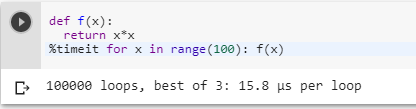3 views
in Python

I understand the concept of what timeit does but I am not sure how to implement it in my code.

How can I compare two functions, say insertion_sort and tim_sort, with timeit?

by (106k points)
edited by

The timeit module can be used in an interactive Python session, there are two convenient options:

The first option is to use the IPython shell. It features the convenient %timeit special function.

def f(x):

return x*x

%timeit for x in range(100): f(x)The second option is to use it in a standard Python interpreter, where you can access functions and other names you defined earlier during the interactive session by importing them from __main__ in the setup statement:

def f(x):

return x * x

import timeit

timeit.repeat("for x in range(100): f(x)", "from __main__ import f", number=100000)by (220 points)
``````>>> import timeit

>>> setup = '''
import random

random.seed('slartibartfast')
s = [random.random() for i in range(1000)]
timsort = list.sort
'''

>>> print min(timeit.Timer('a=s[:]; timsort(a)', setup=setup).repeat(7, 1000))
0.334147930145``````